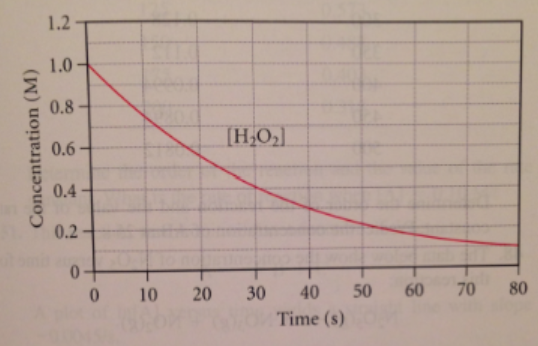# Problem: Consider the reaction:2 H2O2(aq) → 2 H2O(l) + O2(g)The graph below shows the concentration of H2O2 as a function of time.Use the graph to calculate each quantity:d.  If the initial volume of the H2O2 is 1.5 L, what total amount of O2 (in moles) is formed in the first 50 seconds of reaction?

###### FREE Expert Solution

We’re being asked to calculate the total amount of O2 (in moles) is formed in the first 50 seconds of reaction

2 H2O2(aq) → 2 H2O(l) + O2(g)

84% (330 ratings)###### Problem Details

Consider the reaction:

2 H2O2(aq) → 2 H2O(l) + O2(g)

The graph below shows the concentration of H2O2 as a function of time.Use the graph to calculate each quantity:

d.  If the initial volume of the H2O2 is 1.5 L, what total amount of O2 (in moles) is formed in the first 50 seconds of reaction?

Frequently Asked Questions

What scientific concept do you need to know in order to solve this problem?

Our tutors have indicated that to solve this problem you will need to apply the Average Rate of Reaction concept. You can view video lessons to learn Average Rate of Reaction. Or if you need more Average Rate of Reaction practice, you can also practice Average Rate of Reaction practice problems.